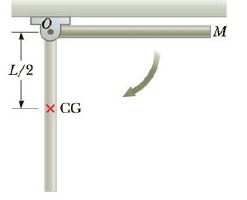Chapter 8, Problem 47P

Chapter
Section
Textbook Problem

The uniform thin rod in Figure P8.47 has mass M = 3.50 kg and length L = 1.00 m and is free to rotate on a friction less pin. At the instant the rod is released from rest in the horizontal position, find the magnitude of (a) the rod’s angular acceleration, (b) the tangential acceleration of the rod’s center of mass, and (c) the tangential acceleration of the rod’s free end.Figure P8.47 Problems 47 and 86.

(a)

To determine
The rod’s angular acceleration.

Explanation

Given info: The mass of the rod is 3.50kg , length of the rod is 1.00m , and acceleration due to gravity is 9.80m/s2 .

Explanation: The net torque of the rod on a frictionless pin is τ=Iα due to the rod’s weight at center of gravity and the moment of inertia of the rod is I=ML2/3 . Now the sum of torques becomes as (L/2)Mg=(ML2/3)α , by rearranging this expression, the angular acceleration is calculated.

The formula for the rod’s angular acceleration is,

α=32gL

• g is acceleration due to gravity

(b)

To determine
The tangential acceleration of the rod’s center of mass.

(c)

To determine
The tangential acceleration of the rod’s free end.

Still sussing out bartleby?

Check out a sample textbook solution.

See a sample solution

The Solution to Your Study Problems

Bartleby provides explanations to thousands of textbook problems written by our experts, many with advanced degrees!

Get Started

Find more solutions based on key concepts